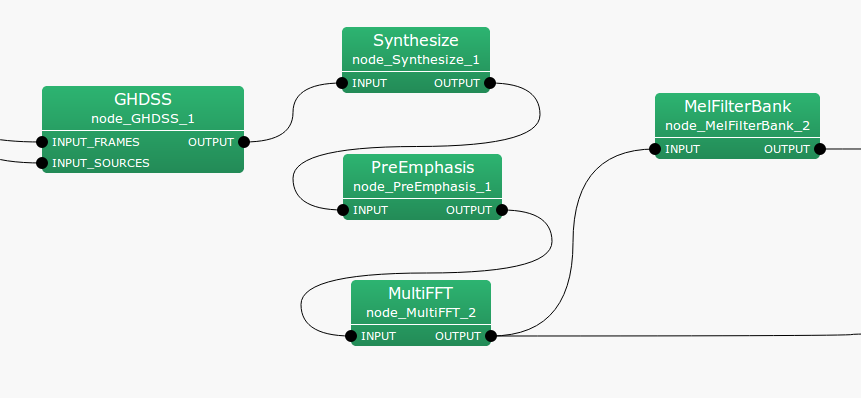## 6.4.6 PreEmphasis

### 6.4.6.1 Outline of the node

This node performs processing to emphasize upper frequency (pre-emphasis) when extracting acoustic features for speech recognition, so as to raise robustness to noise.

### 6.4.6.2 Necessary files

No files are required.

### 6.4.6.3 Usage

This node is generally used before extracting MFCC features. Moreover, it can be used as preprocessing when extracting MSLS features generally used for HARK.

Typical connectionFigure 6.78: Connection example of PreEmphasis

### 6.4.6.4 Input-output and property of the node

Table 6.66: Parameter list of PreEmphasis
 Parameter name Type Default value Unit Description LENGTH 512 [pt] Signal length or window length of FFT SAMPLING_RATE 16000 [Hz] Sampling rate PREEMCOEF 0.97 Preemphasis coefficient INPUT_TYPE WAV Input signal type

Input

INPUT

: Map<int, ObjectRef> , When input signals are time domain waveforms, ObjectRef points to a Vector<float> . If the signals are in the frequency domain, it points to a Vector<complex<float> > .

Output

OUTPUT

: Map<int, ObjectRef> , Signals for which the upper frequency is emphasized. The output corresponds to the type of input; ObjectRef refers to Vector<float> for time domain waveforms and to Vector<complex<float> > for frequency domain signals.

Parameter

LENGTH

When INPUT_TYPE is SPECTRUM, LENGTH indicates FFT length and must be equal to the value set in previous nodes. When INPUT_TYPE is WAV, it indicates the length of the signal contained in one frame and must be equal to the value set in previous nodes. Typically the signal length is same as FFT length.

SAMPLING_RATE

Similar to LENGTH, it is necessary to make this equal to the value in other nodes.

PREEMCOEF

A pre-emphasis coefficient expressed as $c_ p$ below. 0.97 is generally used for speech recognition.

INPUT_TYPE

Two input types of WAV and SPECTRUM are available. WAV is used for time domain waveform inputs. Moreover, SPECTRUM is used for frequency domain signal inputs.

### 6.4.6.5 Details of the node

The necessity and effects of pre-emphasis on common speech recognition are described in various books and theses. Although it is commonly said that this processing makes the system robust to noise, not much performance difference is obtained with this processing with HARK. This is probably because microphone array processing is performed with HARK. It is necessary to make the audio data parameters equal to those used for the speech recognition acoustic model. In other words, when pre-emphasis is performed for the data used for learning acoustic model, the performance is improved by performing pre-emphasis also for input data. Concretely, PreEmphasis consists of two types of processing depending on the type of input signal.

Upper frequency emphasis in time domain:

In the case of time domain, assuming $t$ is the index indicating a sample in a frame, input signals are $s[t]$, the signal for which upper frequency is emphasized is $p[t]$ and the pre-emphasis coefficient is $c_ p$, the upper frequency emphasis in time domain is expressed as follows.

 \begin{equation} \label{eq:pre-time} p[t]= \left\{ \begin{array}{@{\, }ll} s[t]- c_ p \cdot s[t-1] & t > 0 \\ (1 - c_ p) \cdot s & t = 0 \\ \end{array} \right. \end{equation} (129)

Upper frequency emphasis in frequency domain:

In order to realize a frequency domain filter equivalent to the time domain filter, a frequency domain spectral filter equivalent to the time domain $p[t]$ is used. Moreover, 0 is set to the low domain (for four bands from the bottom) and high domain (more than $fs$/2 -100Hz) considering errors. Here, $fs$ indicates sampling frequency.